Скачать презентацию PERCENTAGE Department of Analytical Skills Content

f5976defdfa454e0f56307fd90ac0c15.ppt

• Количество слайдов: 61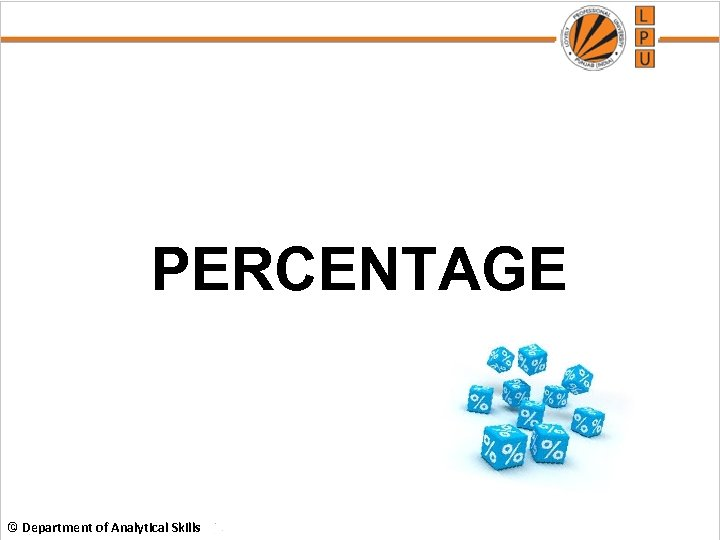PERCENTAGE © Department of Analytical Skills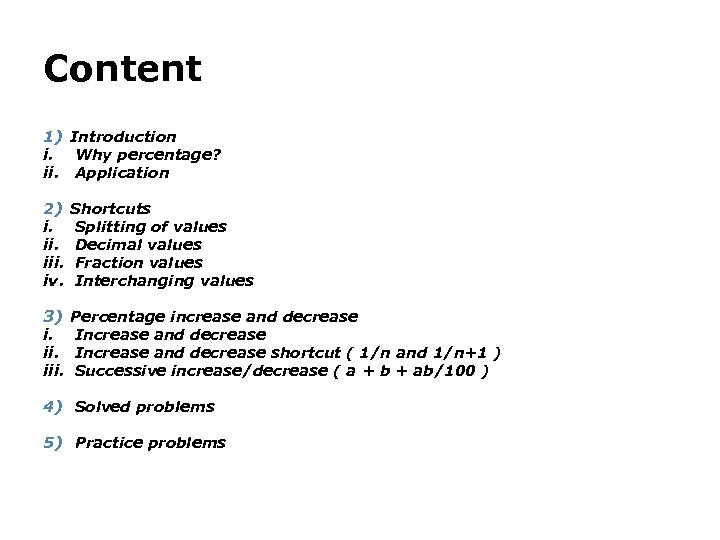Content 1) Introduction i. Why percentage? ii. Application 2) i. iii. iv. Shortcuts Splitting of values Decimal values Fraction values Interchanging values 3) i. iii. Percentage increase and decrease Increase and decrease shortcut ( 1/n and 1/n+1 ) Successive increase/decrease ( a + b + ab/100 ) 4) Solved problems 5) Practice problems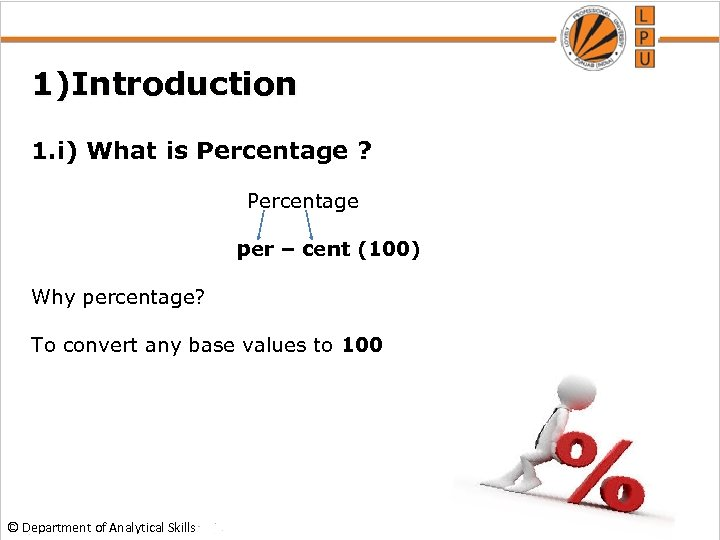1)Introduction 1. i) What is Percentage ? Percentage per – cent (100) Why percentage? To convert any base values to 100 © Department of Analytical Skills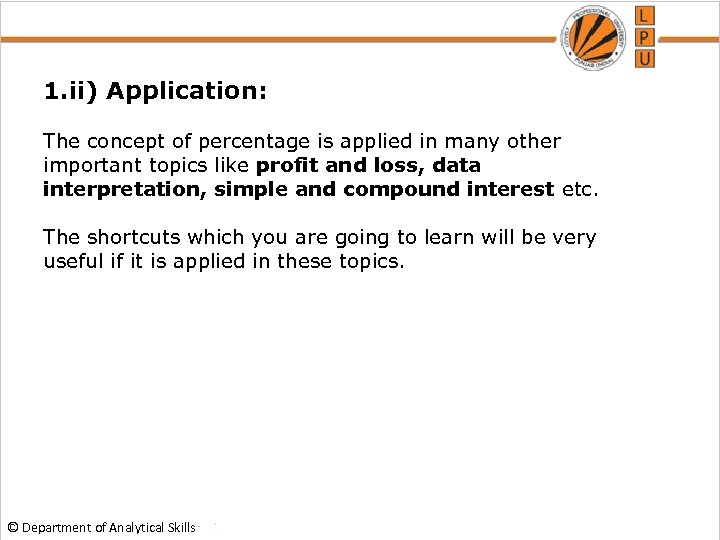1. ii) Application: The concept of percentage is applied in many other important topics like profit and loss, data interpretation, simple and compound interest etc. The shortcuts which you are going to learn will be very useful if it is applied in these topics. © Department of Analytical Skills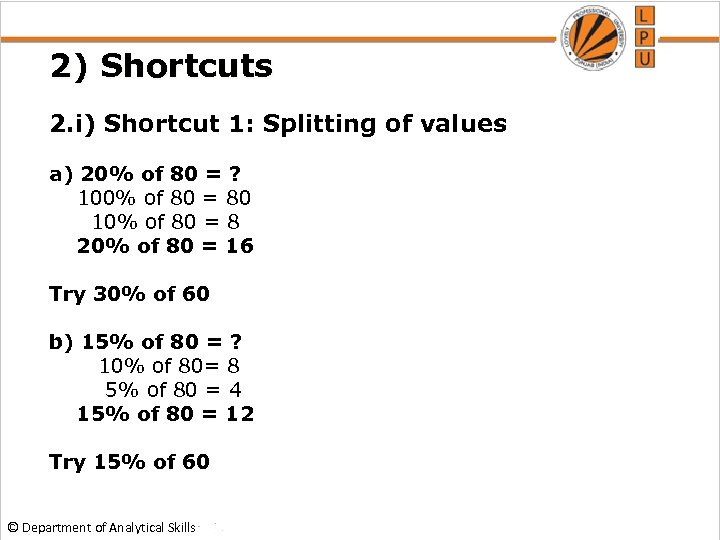2) Shortcuts 2. i) Shortcut 1: Splitting of values a) 20% of 80 = ? 100% of 80 = 80 10% of 80 = 8 20% of 80 = 16 Try 30% of 60 b) 15% of 80 = ? 10% of 80= 8 5% of 80 = 4 15% of 80 = 12 Try 15% of 60 © Department of Analytical Skills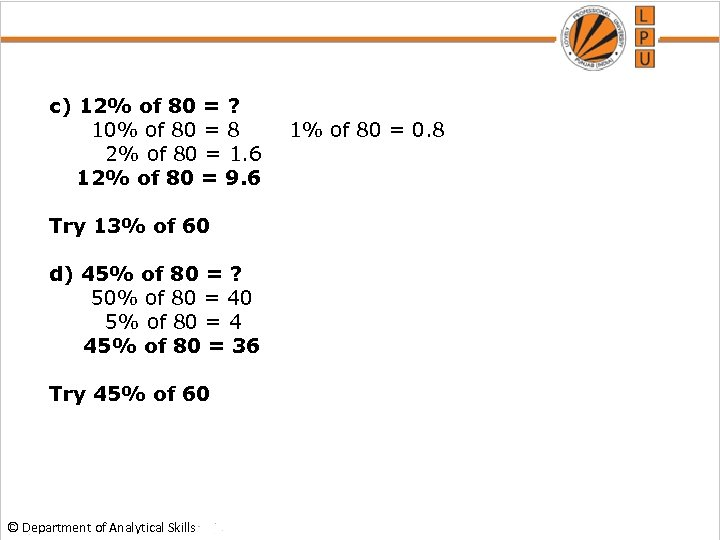c) 12% of 80 = ? 10% of 80 = 8 1% of 80 = 0. 8 2% of 80 = 1. 6 12% of 80 = 9. 6 Try 13% of 60 d) 45% of 80 = ? 50% of 80 = 40 5% of 80 = 4 45% of 80 = 36 Try 45% of 60 © Department of Analytical Skills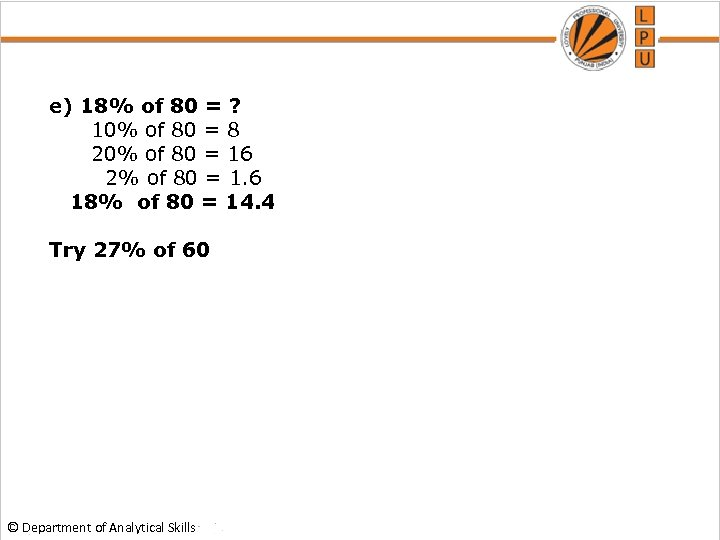e) 18% of 80 = ? 10% of 80 = 8 20% of 80 = 16 2% of 80 = 1. 6 18% of 80 = 14. 4 Try 27% of 60 © Department of Analytical Skills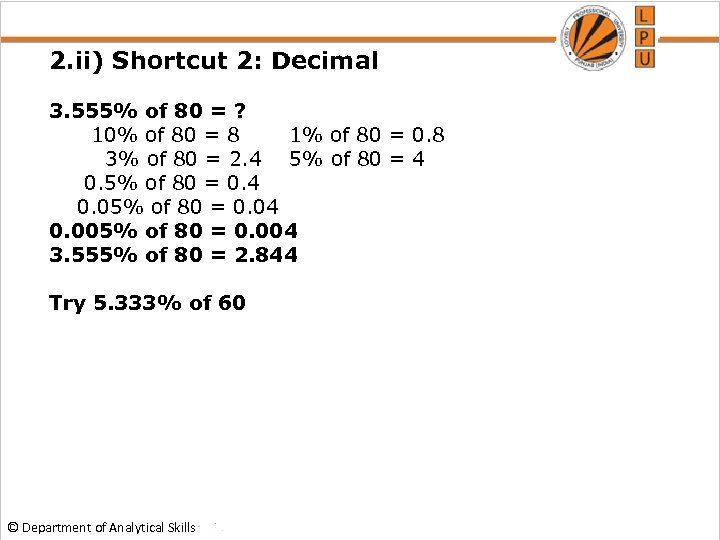2. ii) Shortcut 2: Decimal 3. 555% of 80 = ? 10% of 80 = 8 1% of 80 = 0. 8 3% of 80 = 2. 4 5% of 80 = 4 0. 5% of 80 = 0. 4 0. 05% of 80 = 0. 04 0. 005% of 80 = 0. 004 3. 555% of 80 = 2. 844 Try 5. 333% of 60 © Department of Analytical Skills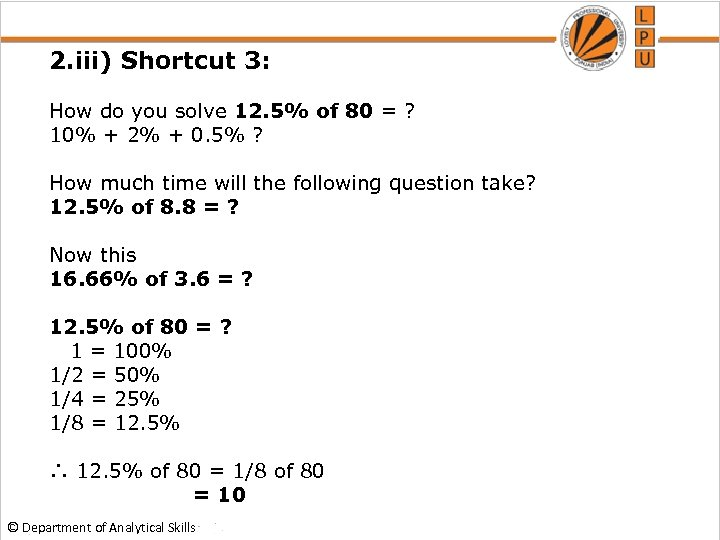2. iii) Shortcut 3: How do you solve 12. 5% of 80 = ? 10% + 2% + 0. 5% ? How much time will the following question take? 12. 5% of 8. 8 = ? Now this 16. 66% of 3. 6 = ? 12. 5% of 80 = ? 1 = 100% 1/2 = 50% 1/4 = 25% 1/8 = 12. 5% ∴ 12. 5% of 80 = 1/8 of 80 = 10 © Department of Analytical Skills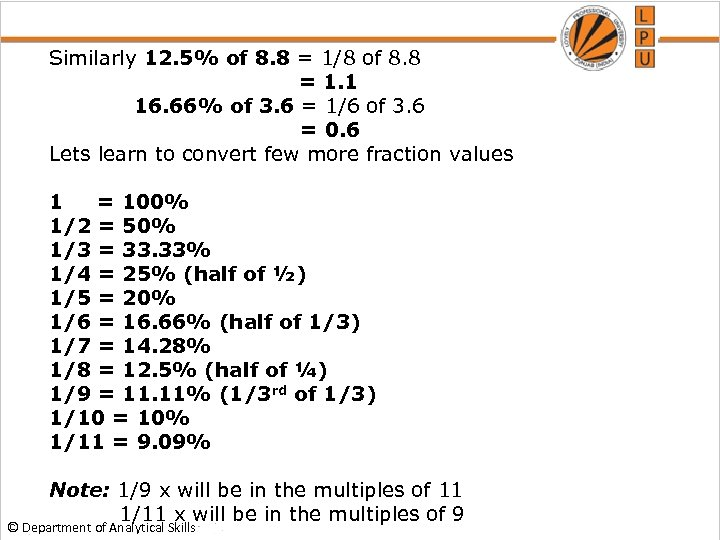Similarly 12. 5% of 8. 8 = 1/8 of 8. 8 = 1. 1 16. 66% of 3. 6 = 1/6 of 3. 6 = 0. 6 Lets learn to convert few more fraction values 1 = 100% 1/2 = 50% 1/3 = 33. 33% 1/4 = 25% (half of ½) 1/5 = 20% 1/6 = 16. 66% (half of 1/3) 1/7 = 14. 28% 1/8 = 12. 5% (half of ¼) 1/9 = 11. 11% (1/3 rd of 1/3) 1/10 = 10% 1/11 = 9. 09% Note: 1/9 x will be in the multiples of 11 1/11 x will be in the multiples of 9 © Department of Analytical Skills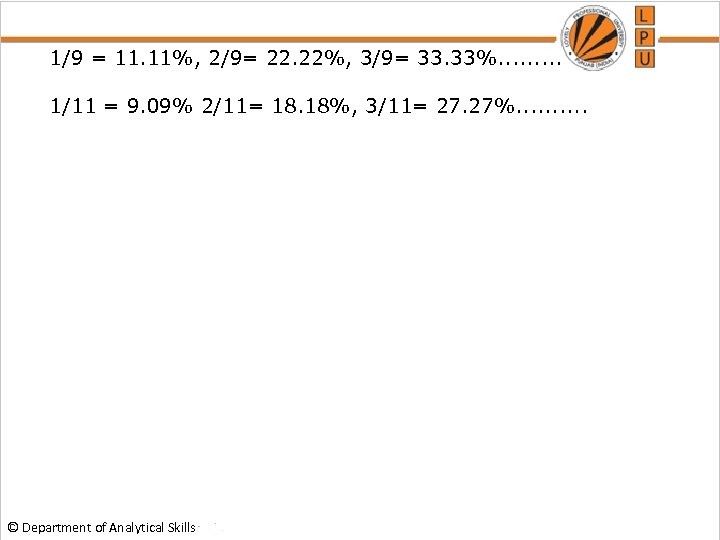1/9 = 11. 11%, 2/9= 22. 22%, 3/9= 33. 33%. . 1/11 = 9. 09% 2/11= 18. 18%, 3/11= 27. 27%. . © Department of Analytical Skills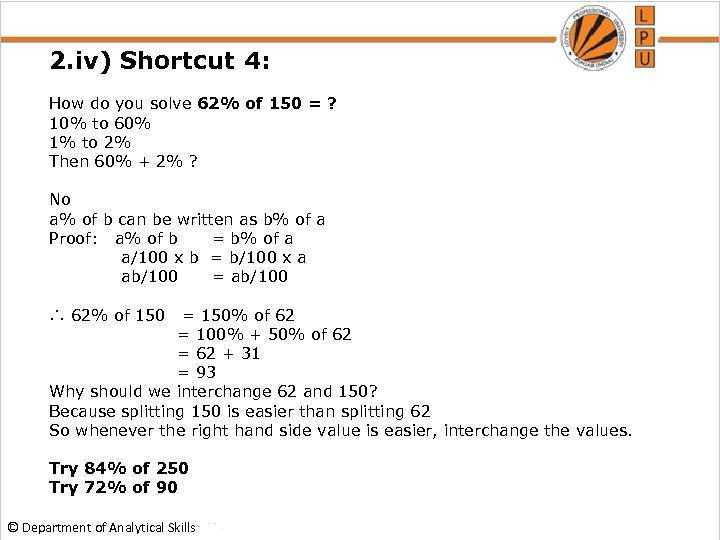2. iv) Shortcut 4: How do you solve 62% of 150 = ? 10% to 60% 1% to 2% Then 60% + 2% ? No a% of b can be written as b% of a Proof: a% of b = b% of a a/100 x b = b/100 x a ab/100 = ab/100 ∴ 62% of 150 = 150% of 62 = 100% + 50% of 62 = 62 + 31 = 93 Why should we interchange 62 and 150? Because splitting 150 is easier than splitting 62 So whenever the right hand side value is easier, interchange the values. Try 84% of 250 Try 72% of 90 © Department of Analytical Skills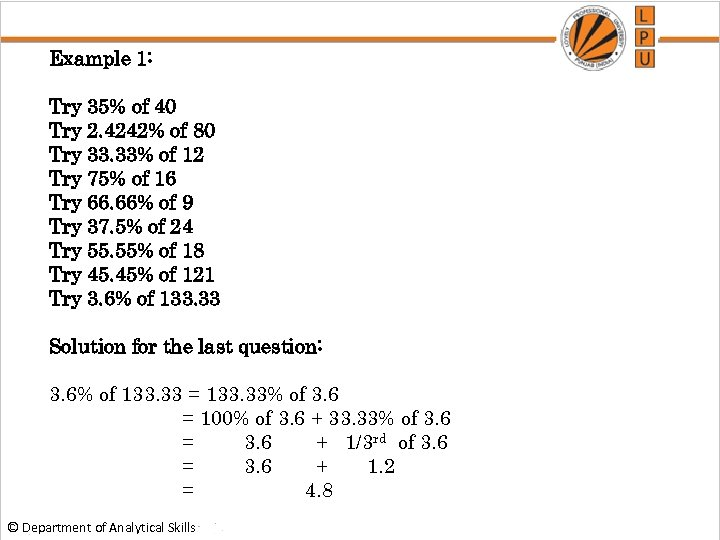Example 1: Try 35% of 40 Try 2. 4242% of 80 Try 33. 33% of 12 Try 75% of 16 Try 66. 66% of 9 Try 37. 5% of 24 Try 55. 55% of 18 Try 45. 45% of 121 Try 3. 6% of 133. 33 Solution for the last question: 3. 6% of 133. 33 = 133. 33% of 3. 6 = 100% of 3. 6 + 33. 33% of 3. 6 = 3. 6 + 1/3 rd of 3. 6 = 3. 6 + 1. 2 = 4. 8 © Department of Analytical Skills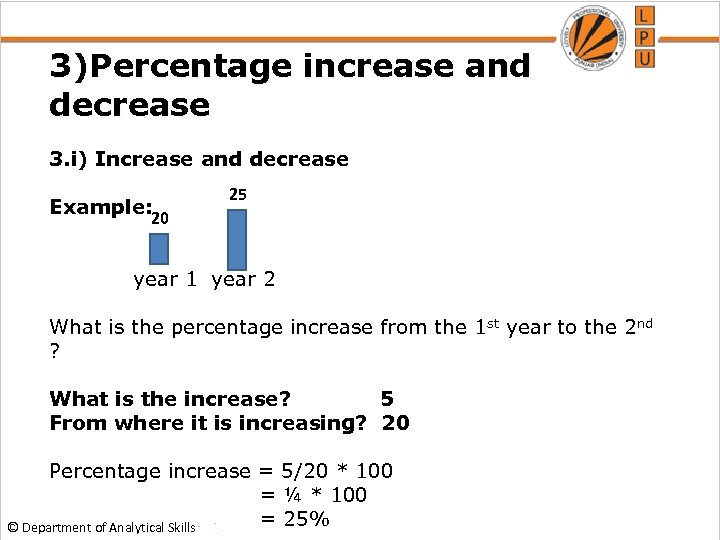3)Percentage increase and decrease 3. i) Increase and decrease Example: 25 20 year 1 year 2 What is the percentage increase from the 1 st year to the 2 nd ? What is the increase? 5 From where it is increasing? 20 Percentage increase = 5/20 * 100 = ¼ * 100 = 25% © Department of Analytical Skills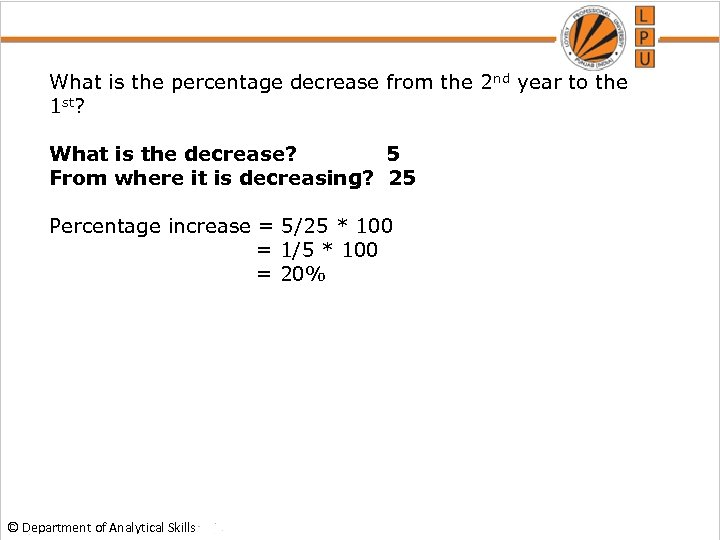What is the percentage decrease from the 2 nd year to the 1 st? What is the decrease? 5 From where it is decreasing? 25 Percentage increase = 5/25 * 100 = 1/5 * 100 = 20% © Department of Analytical Skills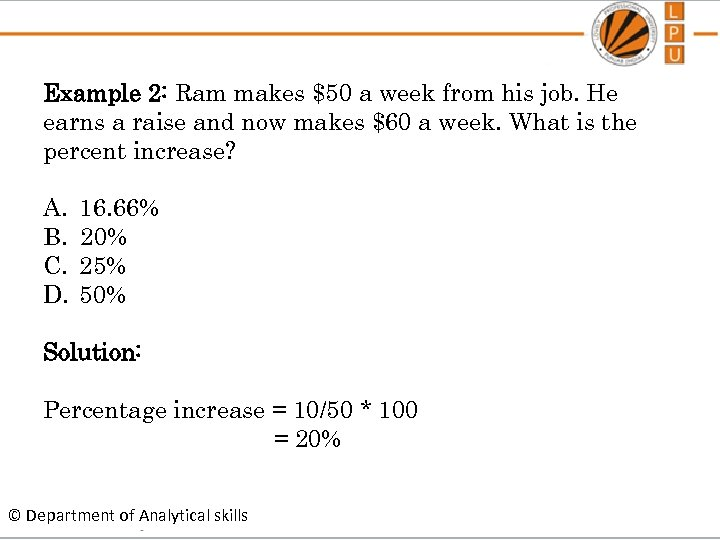Example 2: Ram makes \$50 a week from his job. He earns a raise and now makes \$60 a week. What is the percent increase? A. B. C. D. 16. 66% 20% 25% 50% Solution: Percentage increase = 10/50 * 100 = 20% © Department of Analytical skills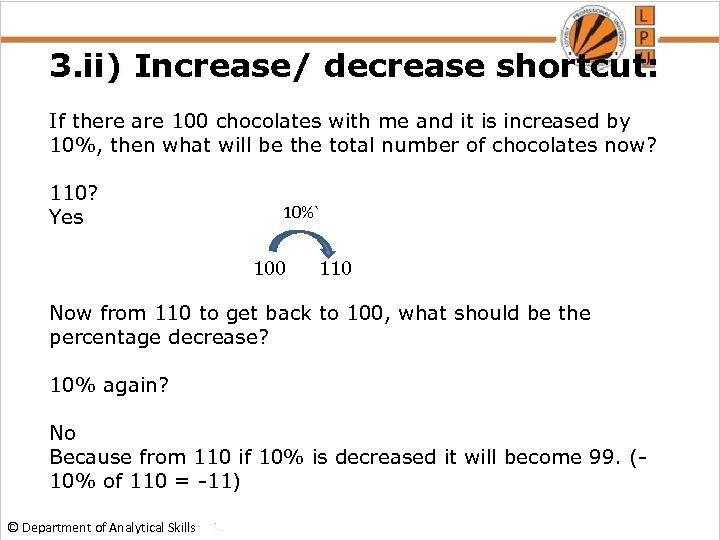3. ii) Increase/ decrease shortcut: If there are 100 chocolates with me and it is increased by 10%, then what will be the total number of chocolates now? 110? 10%` Yes 100 110 Now from 110 to get back to 100, what should be the percentage decrease? 10% again? No Because from 110 if 10% is decreased it will become 99. (10% of 110 = -11) © Department of Analytical Skills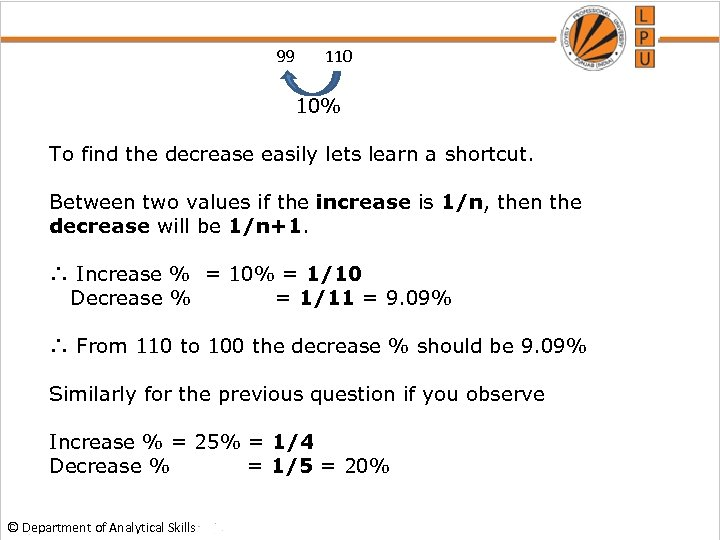99 110 10% To find the decrease easily lets learn a shortcut. Between two values if the increase is 1/n, then the decrease will be 1/n+1. ∴ Increase % = 10% = 1/10 Decrease % = 1/11 = 9. 09% ∴ From 110 to 100 the decrease % should be 9. 09% Similarly for the previous question if you observe Increase % = 25% = 1/4 Decrease % = 1/5 = 20% © Department of Analytical Skills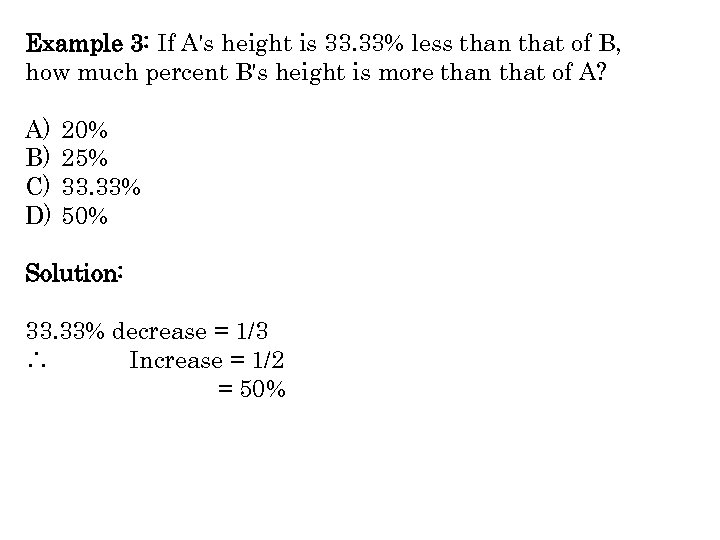Example 3: If A's height is 33. 33% less than that of B, how much percent B's height is more than that of A? A) B) C) D) 20% 25% 33. 33% 50% Solution: 33. 33% decrease = 1/3 ∴ Increase = 1/2 = 50%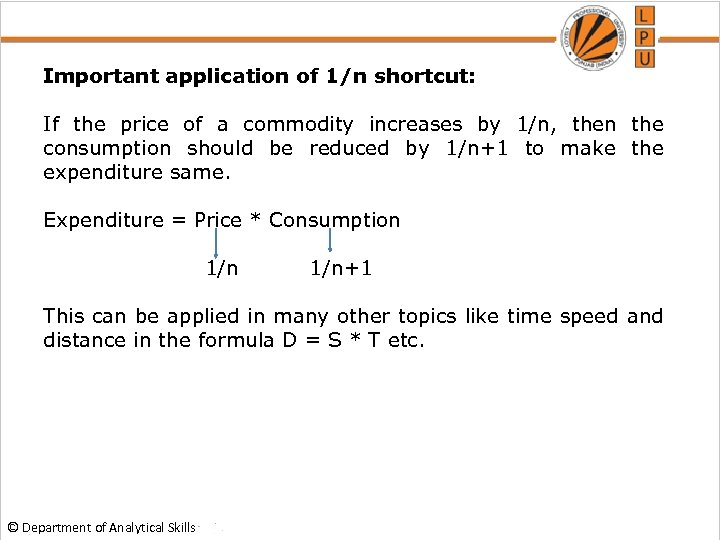Important application of 1/n shortcut: If the price of a commodity increases by 1/n, then the consumption should be reduced by 1/n+1 to make the expenditure same. Expenditure = Price * Consumption 1/n+1 This can be applied in many other topics like time speed and distance in the formula D = S * T etc. © Department of Analytical Skills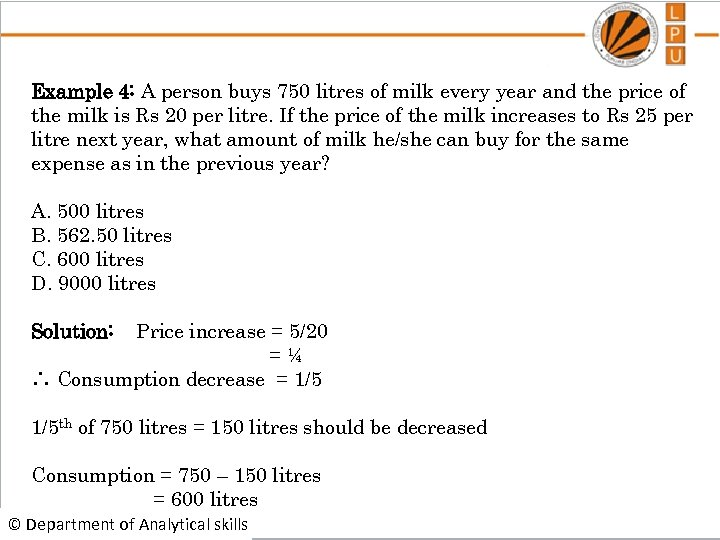Example 4: A person buys 750 litres of milk every year and the price of the milk is Rs 20 per litre. If the price of the milk increases to Rs 25 per litre next year, what amount of milk he/she can buy for the same expense as in the previous year? A. 500 litres B. 562. 50 litres C. 600 litres D. 9000 litres Solution: Price increase = 5/20 =¼ ∴ Consumption decrease = 1/5 th of 750 litres = 150 litres should be decreased Consumption = 750 – 150 litres = 600 litres © Department of Analytical skills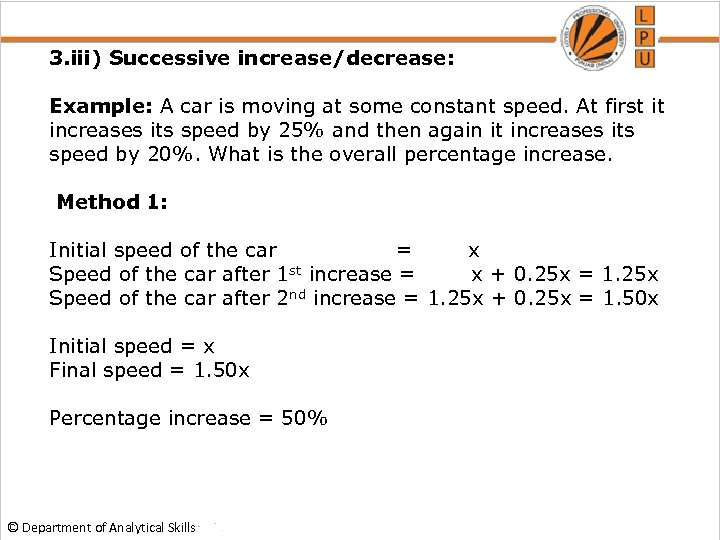3. iii) Successive increase/decrease: Example: A car is moving at some constant speed. At first it increases its speed by 25% and then again it increases its speed by 20%. What is the overall percentage increase. Method 1: Initial speed of the car = x Speed of the car after 1 st increase = x + 0. 25 x = 1. 25 x Speed of the car after 2 nd increase = 1. 25 x + 0. 25 x = 1. 50 x Initial speed = x Final speed = 1. 50 x Percentage increase = 50% © Department of Analytical Skills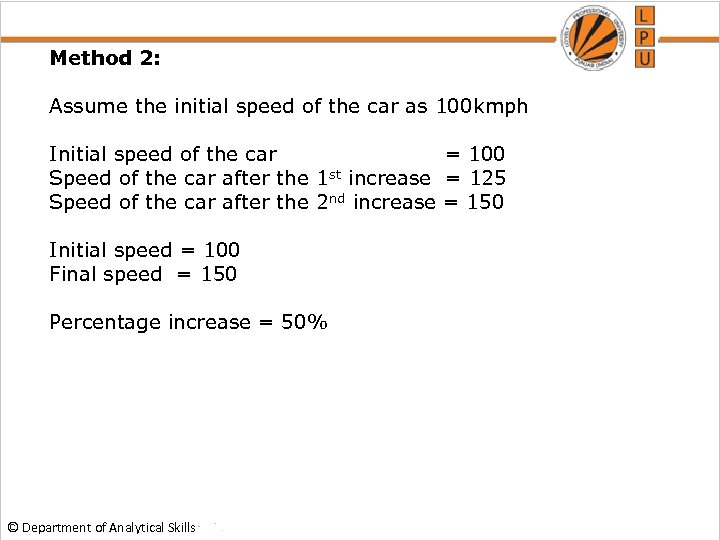Method 2: Assume the initial speed of the car as 100 kmph Initial speed of the car = 100 Speed of the car after the 1 st increase = 125 Speed of the car after the 2 nd increase = 150 Initial speed = 100 Final speed = 150 Percentage increase = 50% © Department of Analytical Skills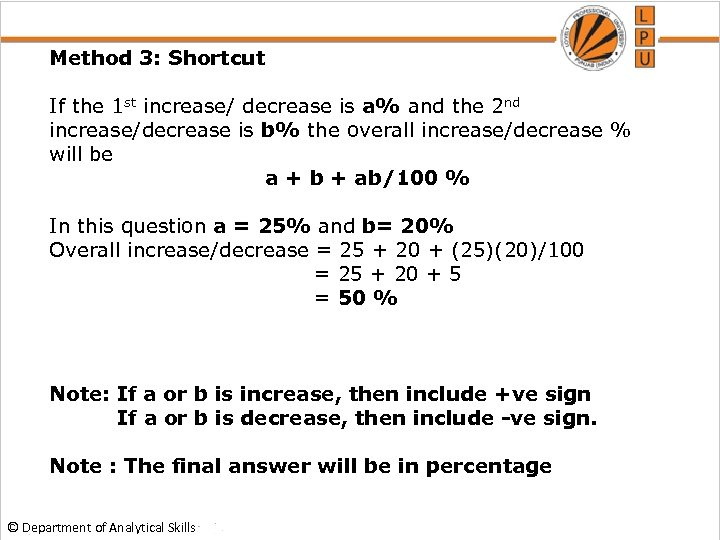Method 3: Shortcut If the 1 st increase/ decrease is a% and the 2 nd increase/decrease is b% the overall increase/decrease % will be a + b + ab/100 % In this question a = 25% and b= 20% Overall increase/decrease = 25 + 20 + (25)(20)/100 = 25 + 20 + 5 = 50 % Note: If a or b is increase, then include +ve sign If a or b is decrease, then include -ve sign. Note : The final answer will be in percentage © Department of Analytical Skills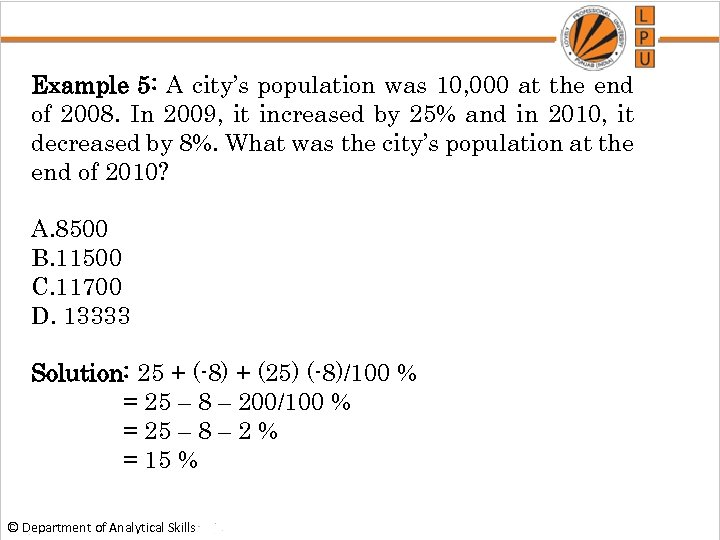Example 5: A city’s population was 10, 000 at the end of 2008. In 2009, it increased by 25% and in 2010, it decreased by 8%. What was the city’s population at the end of 2010? A. 8500 B. 11500 C. 11700 D. 13333 Solution: 25 + (-8) + (25) (-8)/100 % = 25 – 8 – 200/100 % = 25 – 8 – 2 % = 15 % © Department of Analytical Skills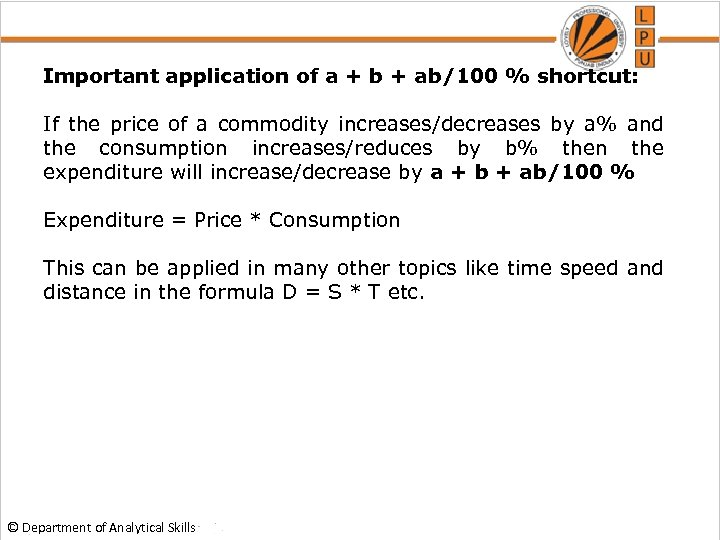Important application of a + b + ab/100 % shortcut: If the price of a commodity increases/decreases by a% and the consumption increases/reduces by b% then the expenditure will increase/decrease by a + b + ab/100 % Expenditure = Price * Consumption This can be applied in many other topics like time speed and distance in the formula D = S * T etc. © Department of Analytical Skills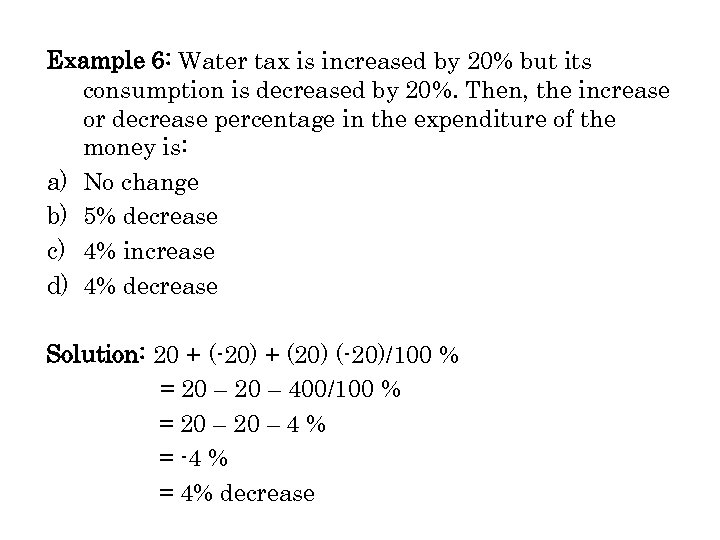Example 6: Water tax is increased by 20% but its consumption is decreased by 20%. Then, the increase or decrease percentage in the expenditure of the money is: a) No change b) 5% decrease c) 4% increase d) 4% decrease Solution: 20 + (-20) + (20) (-20)/100 % = 20 – 400/100 % = 20 – 4 % = -4 % = 4% decrease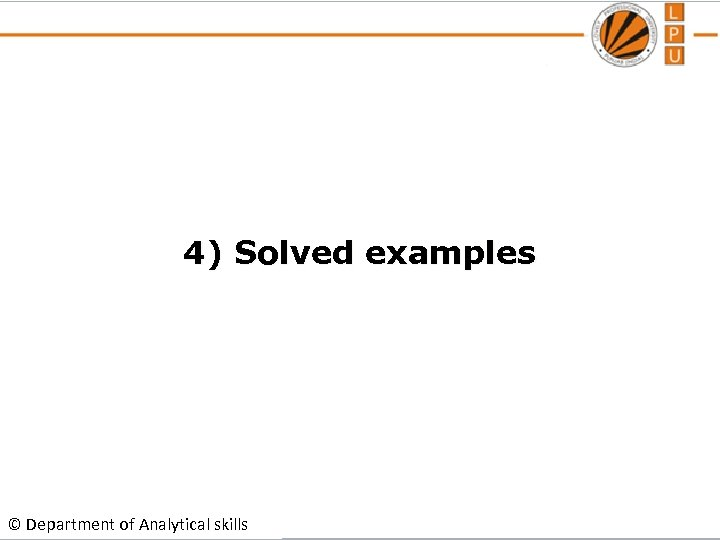4) Solved examples © Department of Analytical skills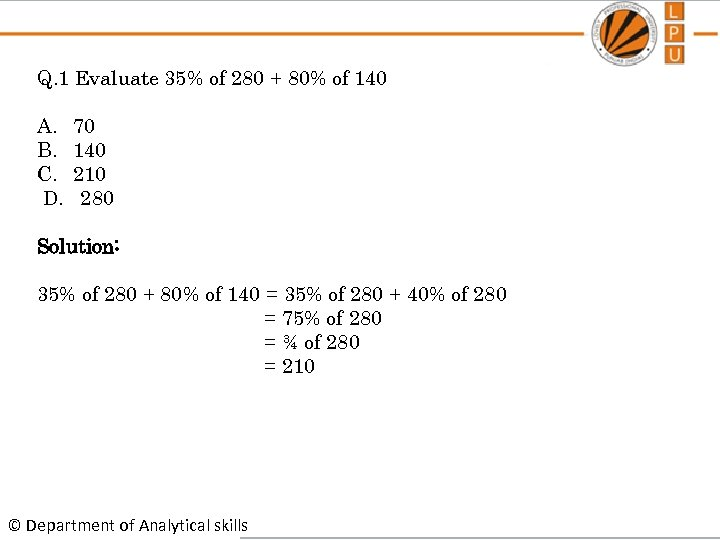Q. 1 Evaluate 35% of 280 + 80% of 140 A. B. C. D. 70 140 210 280 Solution: 35% of 280 + 80% of 140 = 35% of 280 + 40% of 280 = 75% of 280 = ¾ of 280 = 210 © Department of Analytical skills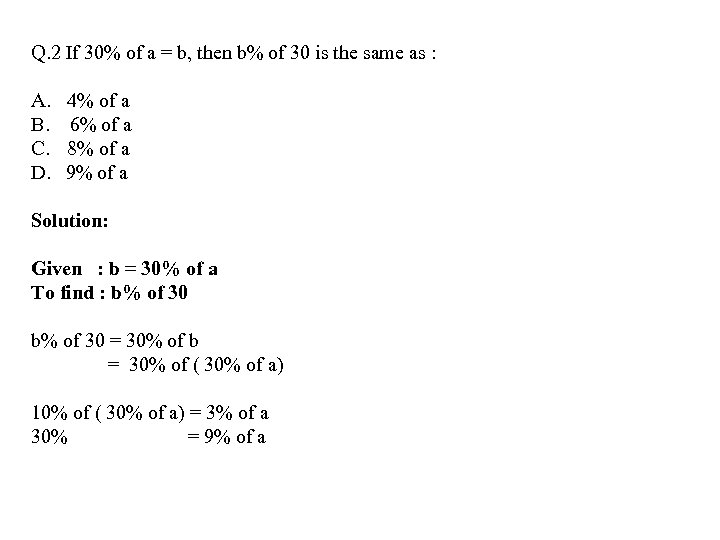Q. 2 If 30% of a = b, then b% of 30 is the same as : A. 4% of a B. 6% of a C. 8% of a D. 9% of a Solution: Given : b = 30% of a To find : b% of 30 = 30% of b = 30% of ( 30% of a) 10% of ( 30% of a) = 3% of a 30% = 9% of a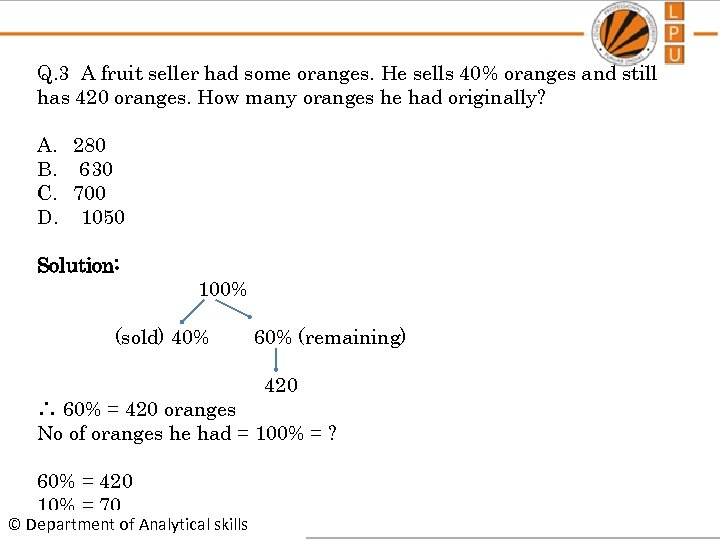Q. 3 A fruit seller had some oranges. He sells 40% oranges and still has 420 oranges. How many oranges he had originally? A. 280 B. 630 C. 700 D. 1050 Solution: 100% (sold) 40% 60% (remaining) 420 ∴ 60% = 420 oranges No of oranges he had = 100% = ? 60% = 420 10% = 70 © Department of Analytical skills 100% = 700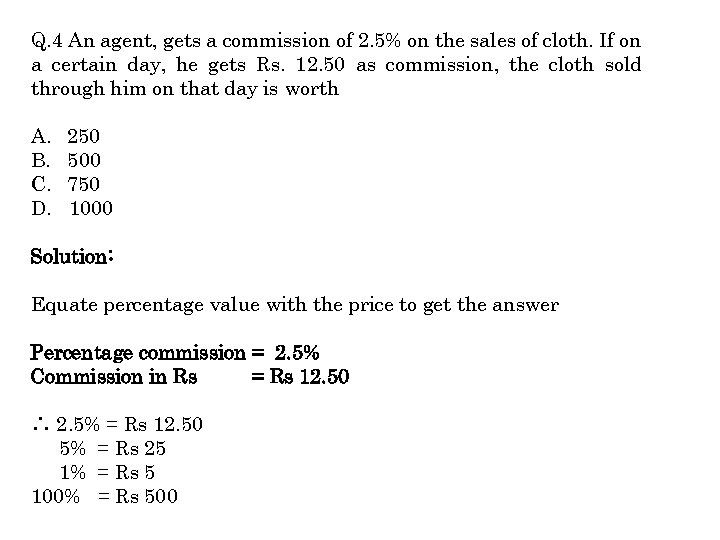Q. 4 An agent, gets a commission of 2. 5% on the sales of cloth. If on a certain day, he gets Rs. 12. 50 as commission, the cloth sold through him on that day is worth A. B. C. D. 250 500 750 1000 Solution: Equate percentage value with the price to get the answer Percentage commission = 2. 5% Commission in Rs = Rs 12. 50 ∴ 2. 5% = Rs 12. 50 5% = Rs 25 1% = Rs 5 100% = Rs 500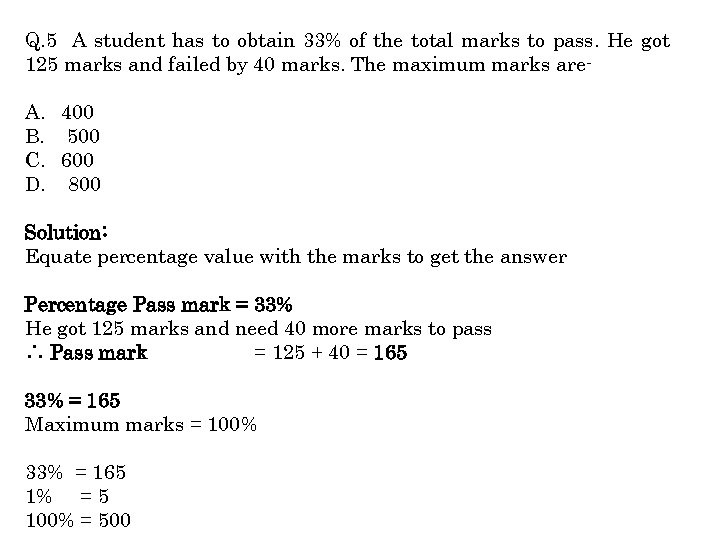Q. 5 A student has to obtain 33% of the total marks to pass. He got 125 marks and failed by 40 marks. The maximum marks are- A. 400 B. 500 C. 600 D. 800 Solution: Equate percentage value with the marks to get the answer Percentage Pass mark = 33% He got 125 marks and need 40 more marks to pass ∴ Pass mark = 125 + 40 = 165 33% = 165 Maximum marks = 100% 33% = 165 1% = 5 100% = 500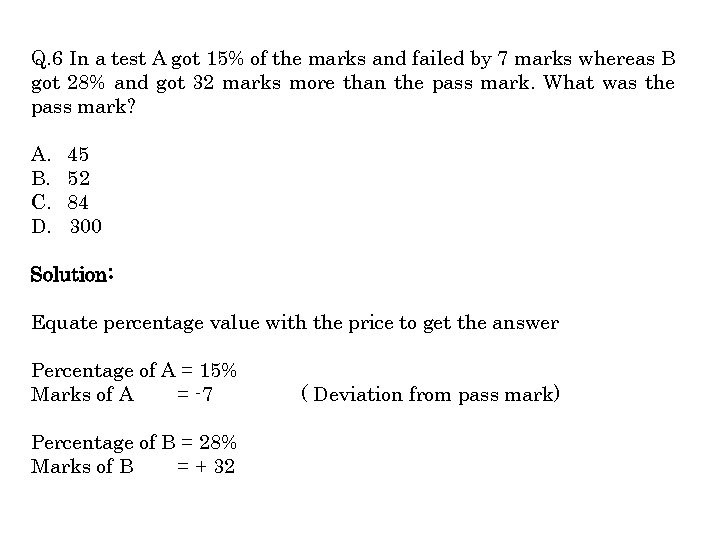Q. 6 In a test A got 15% of the marks and failed by 7 marks whereas B got 28% and got 32 marks more than the pass mark. What was the pass mark? A. B. C. D. 45 52 84 300 Solution: Equate percentage value with the price to get the answer Percentage of A = 15% Marks of A = -7 Percentage of B = 28% Marks of B = + 32 ( Deviation from pass mark)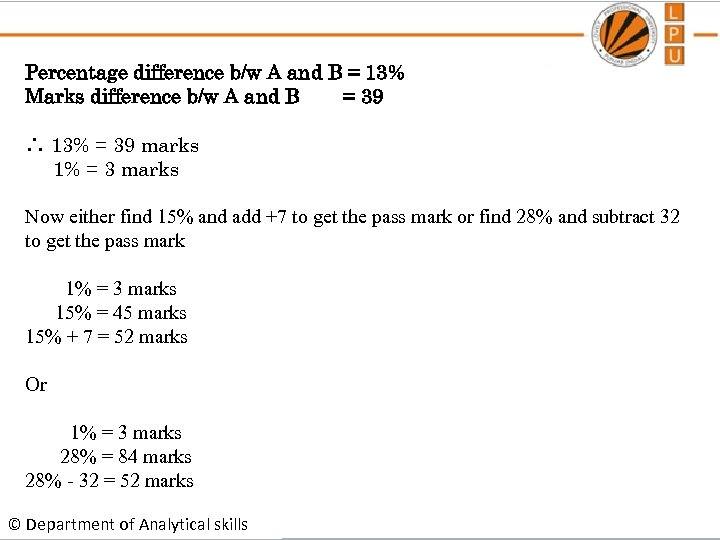Percentage difference b/w A and B = 13% Marks difference b/w A and B = 39 ∴ 13% = 39 marks 1% = 3 marks Now either find 15% and add +7 to get the pass mark or find 28% and subtract 32 to get the pass mark 1% = 3 marks 15% = 45 marks 15% + 7 = 52 marks Or 1% = 3 marks 28% = 84 marks 28% - 32 = 52 marks © Department of Analytical skills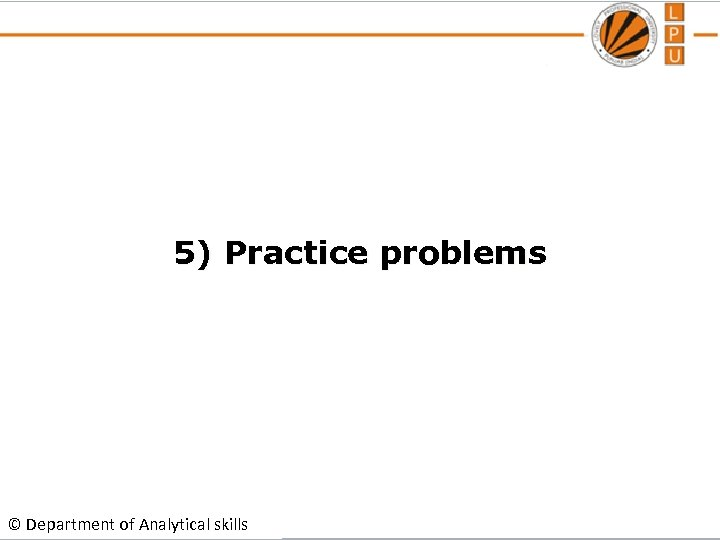5) Practice problems © Department of Analytical skills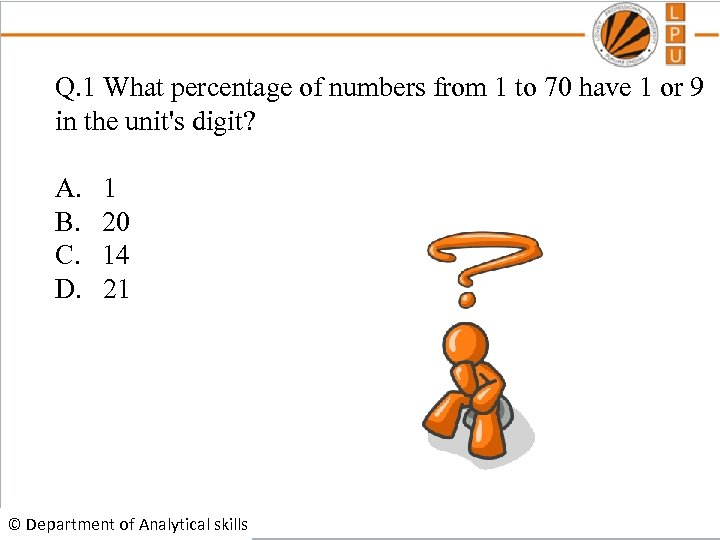Q. 1 What percentage of numbers from 1 to 70 have 1 or 9 in the unit's digit? A. 1 B. 20 C. 14 D. 21 © Department of Analytical skills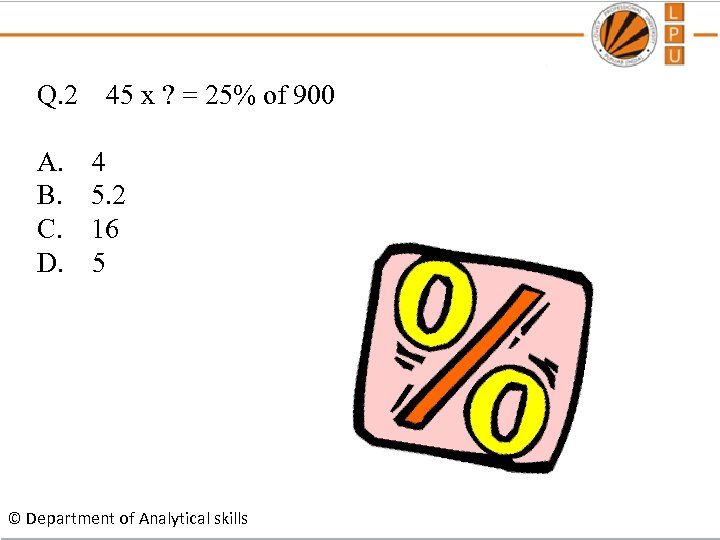Q. 2 45 x ? = 25% of 900 A. 4 B. 5. 2 C. 16 D. 5 © Department of Analytical skills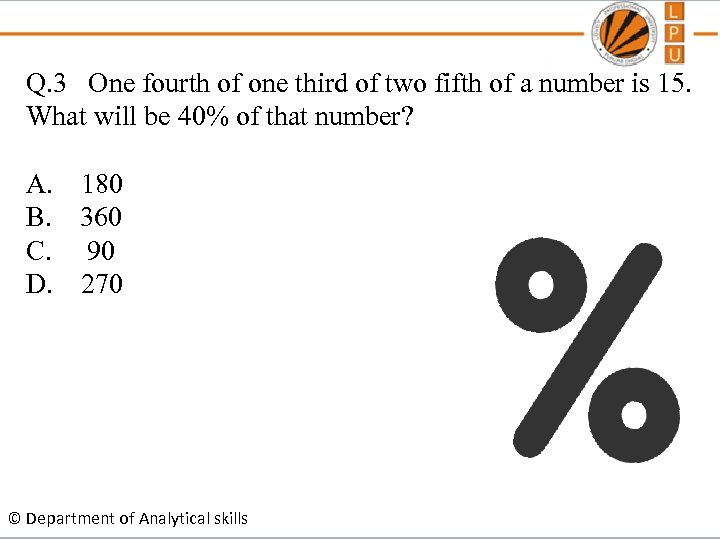Q. 3 One fourth of one third of two fifth of a number is 15. What will be 40% of that number? A. 180 B. 360 C. 90 D. 270 © Department of Analytical skills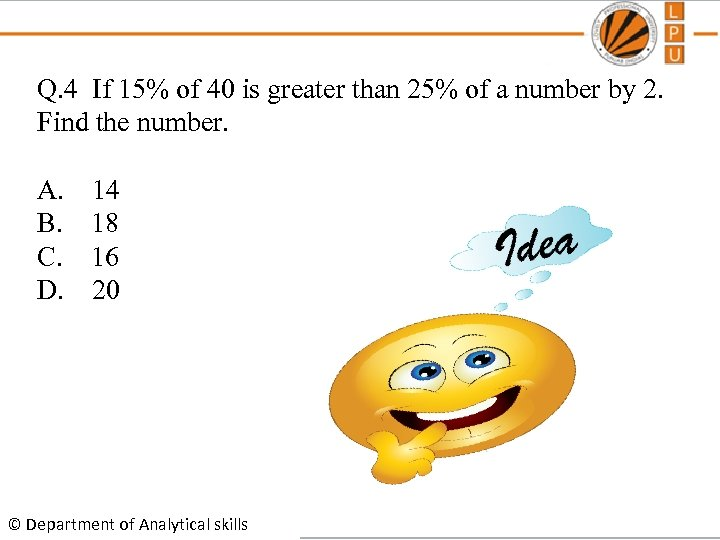Q. 4 If 15% of 40 is greater than 25% of a number by 2. Find the number. A. 14 B. 18 C. 16 D. 20 © Department of Analytical skills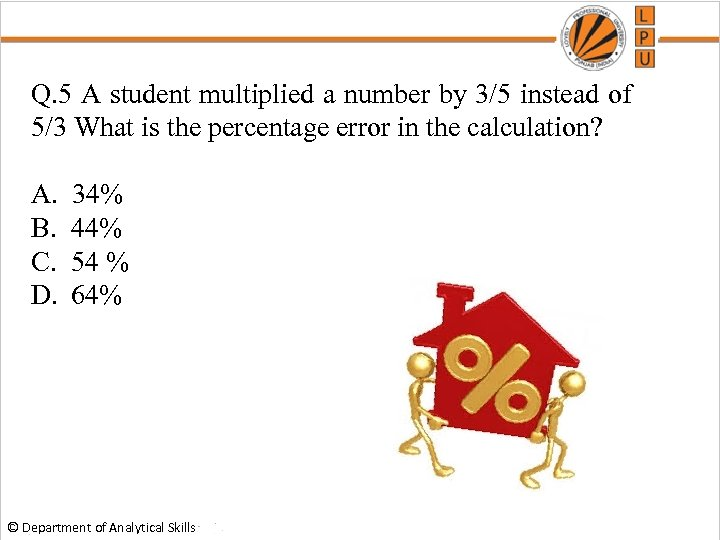Q. 5 A student multiplied a number by 3/5 instead of 5/3 What is the percentage error in the calculation? A. 34% B. 44% C. 54 % D. 64% © Department of Analytical Skills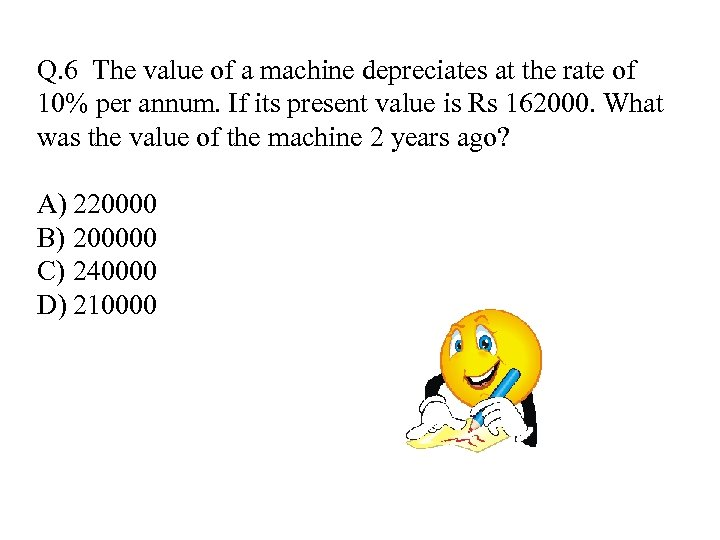Q. 6 The value of a machine depreciates at the rate of 10% per annum. If its present value is Rs 162000. What was the value of the machine 2 years ago? A) 220000 B) 200000 C) 240000 D) 210000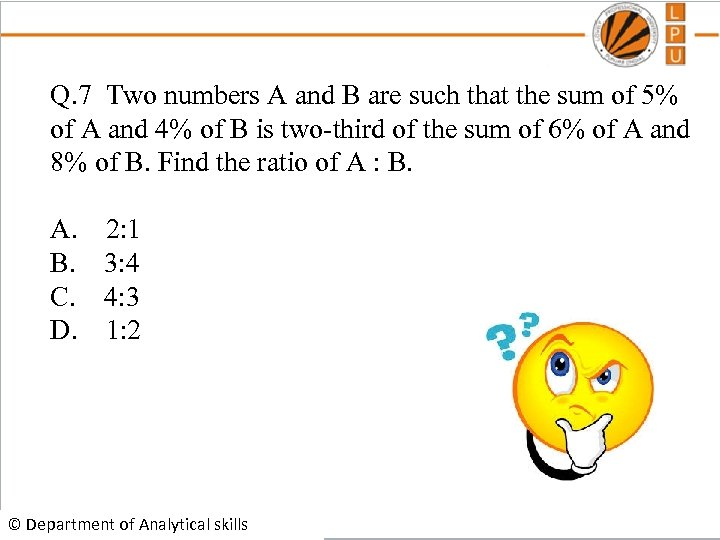Q. 7 Two numbers A and B are such that the sum of 5% of A and 4% of B is two-third of the sum of 6% of A and 8% of B. Find the ratio of A : B. A. 2: 1 B. 3: 4 C. 4: 3 D. 1: 2 © Department of Analytical skills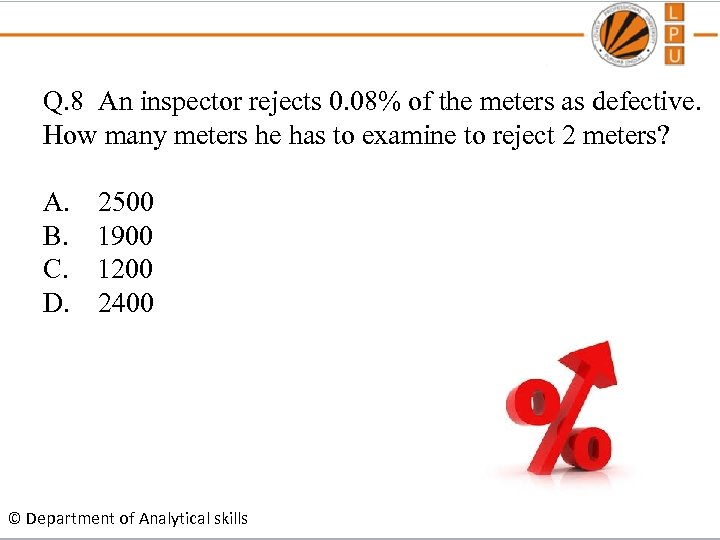Q. 8 An inspector rejects 0. 08% of the meters as defective. How many meters he has to examine to reject 2 meters? A. 2500 B. 1900 C. 1200 D. 2400 © Department of Analytical skills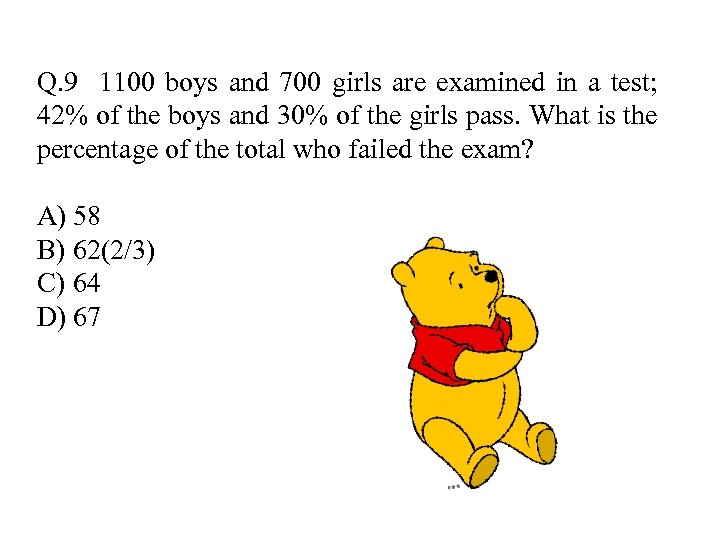Q. 9 1100 boys and 700 girls are examined in a test; 42% of the boys and 30% of the girls pass. What is the percentage of the total who failed the exam? A) 58 B) 62(2/3) C) 64 D) 67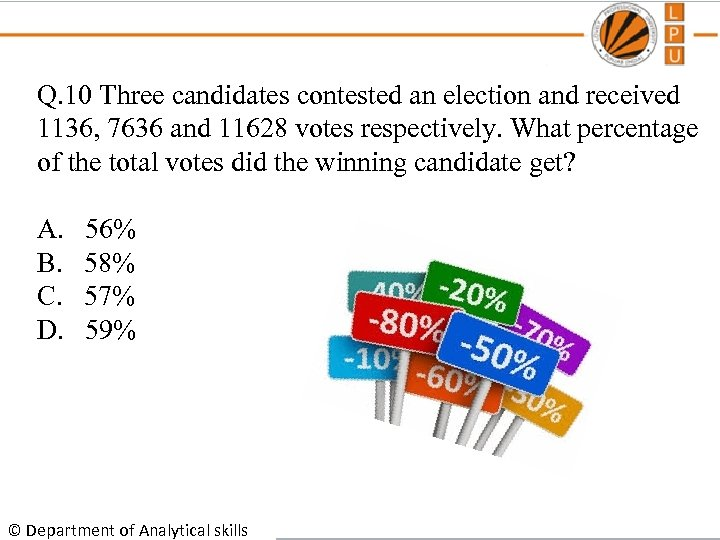Q. 10 Three candidates contested an election and received 1136, 7636 and 11628 votes respectively. What percentage of the total votes did the winning candidate get? A. 56% B. 58% C. 57% D. 59% © Department of Analytical skills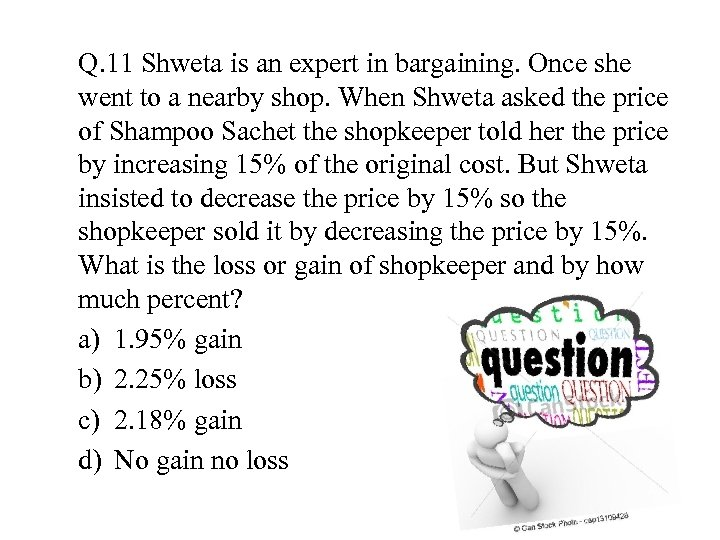Q. 11 Shweta is an expert in bargaining. Once she went to a nearby shop. When Shweta asked the price of Shampoo Sachet the shopkeeper told her the price by increasing 15% of the original cost. But Shweta insisted to decrease the price by 15% so the shopkeeper sold it by decreasing the price by 15%. What is the loss or gain of shopkeeper and by how much percent? a) 1. 95% gain b) 2. 25% loss c) 2. 18% gain d) No gain no loss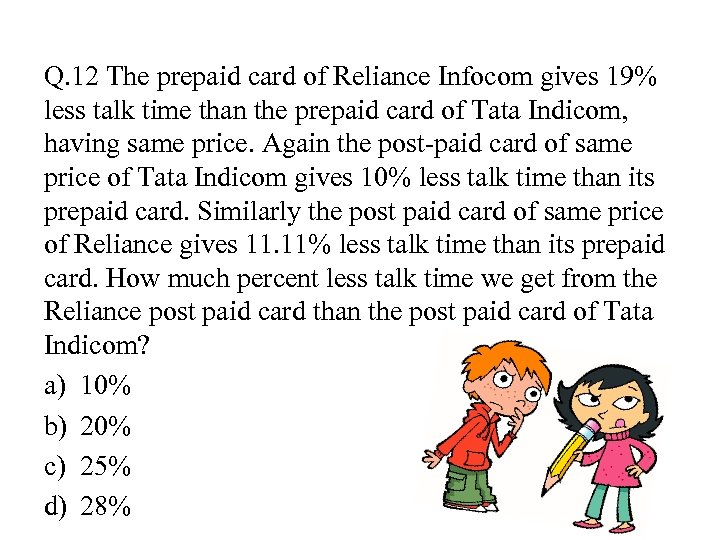Q. 12 The prepaid card of Reliance Infocom gives 19% less talk time than the prepaid card of Tata Indicom, having same price. Again the post-paid card of same price of Tata Indicom gives 10% less talk time than its prepaid card. Similarly the post paid card of same price of Reliance gives 11. 11% less talk time than its prepaid card. How much percent less talk time we get from the Reliance post paid card than the post paid card of Tata Indicom? a) 10% b) 20% c) 25% d) 28%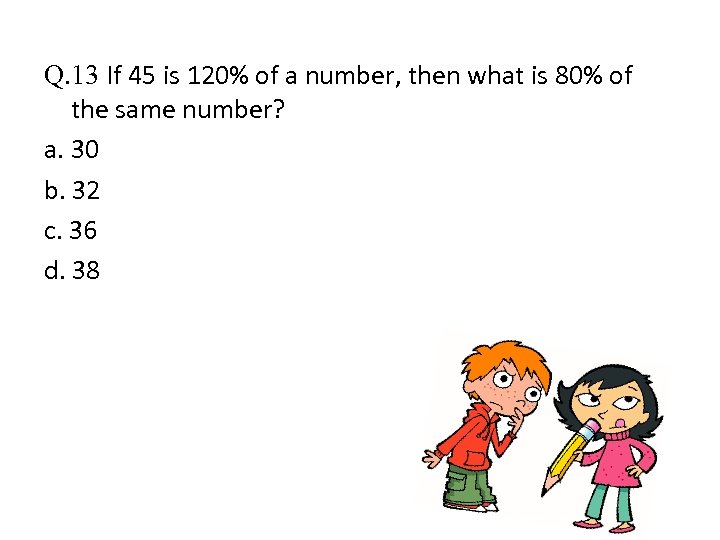Q. 13 If 45 is 120% of a number, then what is 80% of the same number? a. 30 b. 32 c. 36 d. 38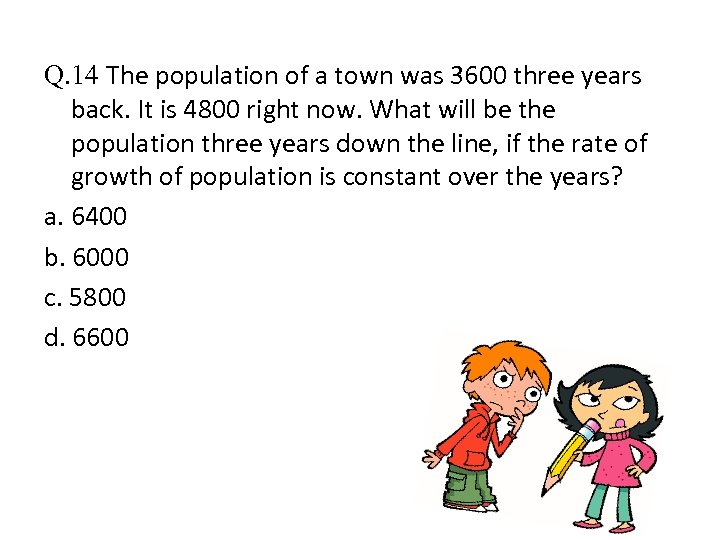Q. 14 The population of a town was 3600 three years back. It is 4800 right now. What will be the population three years down the line, if the rate of growth of population is constant over the years? a. 6400 b. 6000 c. 5800 d. 6600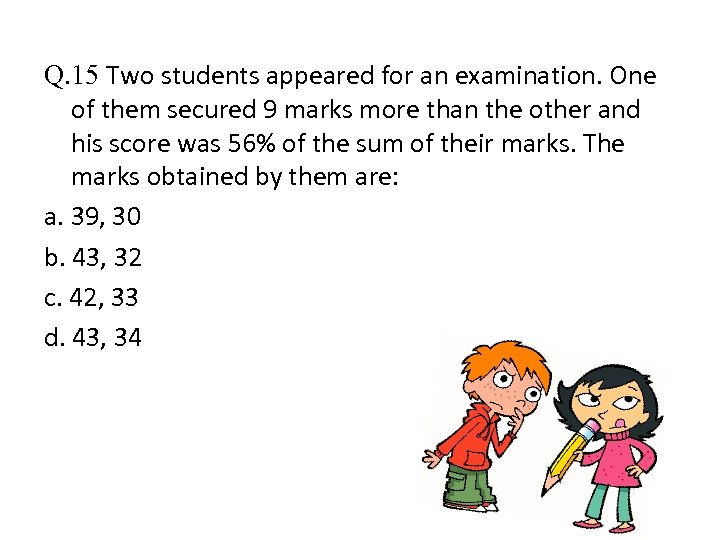Q. 15 Two students appeared for an examination. One of them secured 9 marks more than the other and his score was 56% of the sum of their marks. The marks obtained by them are: a. 39, 30 b. 43, 32 c. 42, 33 d. 43, 34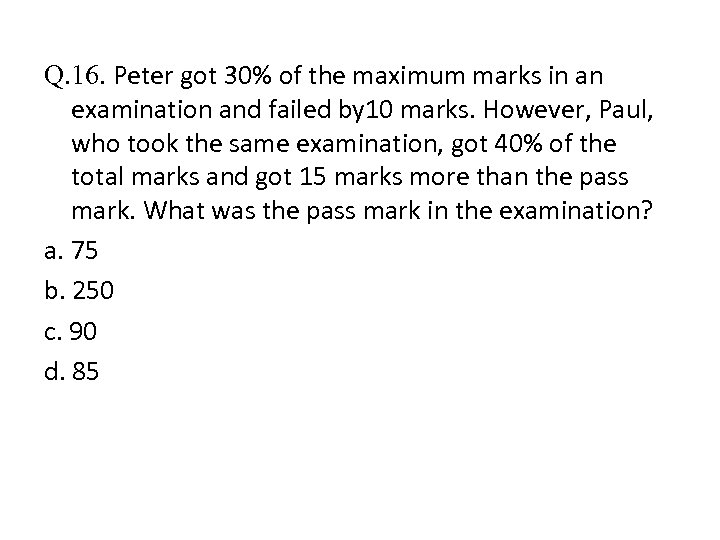Q. 16. Peter got 30% of the maximum marks in an examination and failed by 10 marks. However, Paul, who took the same examination, got 40% of the total marks and got 15 marks more than the pass mark. What was the pass mark in the examination? a. 75 b. 250 c. 90 d. 85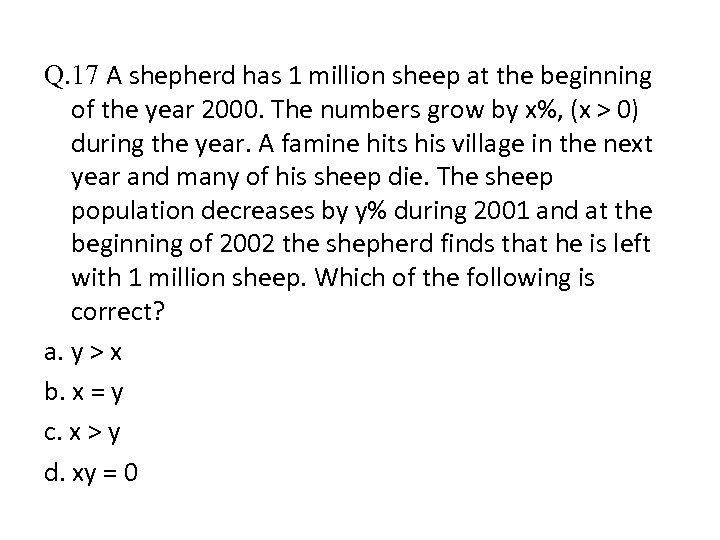Q. 17 A shepherd has 1 million sheep at the beginning of the year 2000. The numbers grow by x%, (x > 0) during the year. A famine hits his village in the next year and many of his sheep die. The sheep population decreases by y% during 2001 and at the beginning of 2002 the shepherd finds that he is left with 1 million sheep. Which of the following is correct? a. y > x b. x = y c. x > y d. xy = 0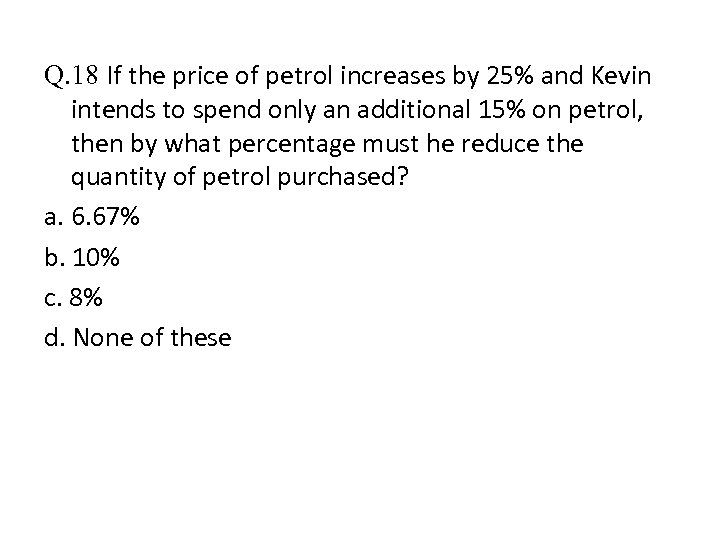Q. 18 If the price of petrol increases by 25% and Kevin intends to spend only an additional 15% on petrol, then by what percentage must he reduce the quantity of petrol purchased? a. 6. 67% b. 10% c. 8% d. None of these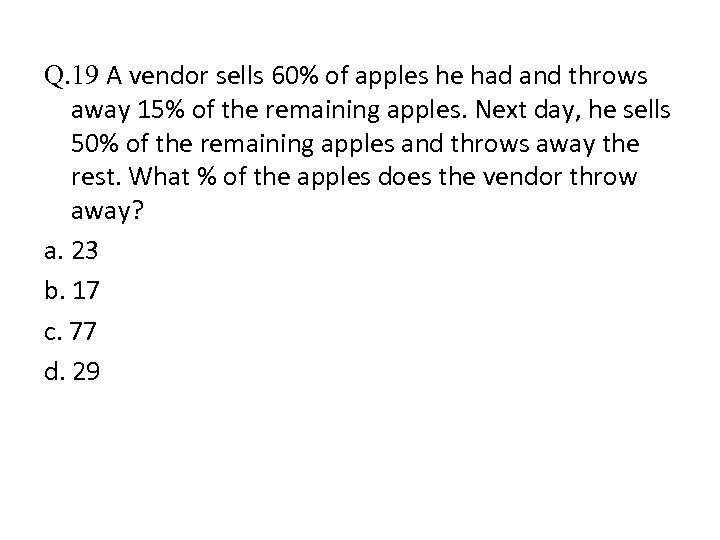Q. 19 A vendor sells 60% of apples he had and throws away 15% of the remaining apples. Next day, he sells 50% of the remaining apples and throws away the rest. What % of the apples does the vendor throw away? a. 23 b. 17 c. 77 d. 29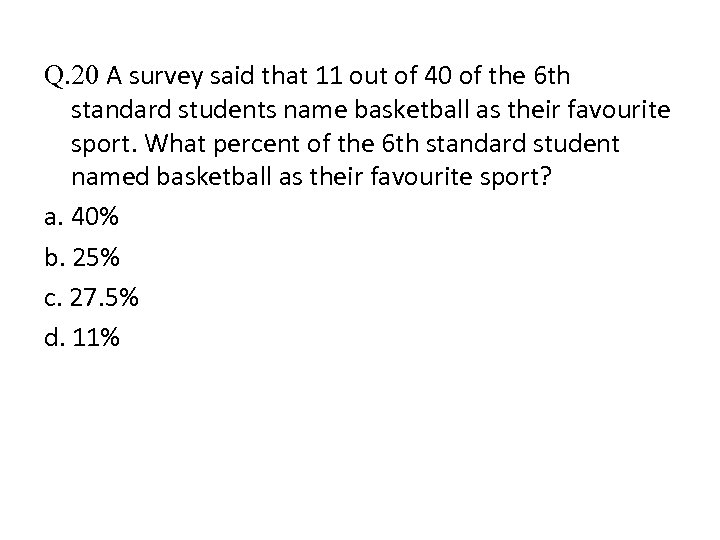Q. 20 A survey said that 11 out of 40 of the 6 th standard students name basketball as their favourite sport. What percent of the 6 th standard student named basketball as their favourite sport? a. 40% b. 25% c. 27. 5% d. 11%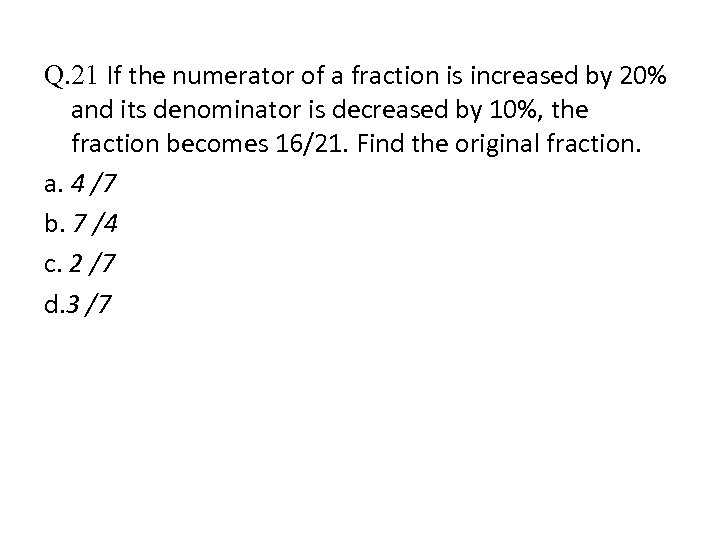Q. 21 If the numerator of a fraction is increased by 20% and its denominator is decreased by 10%, the fraction becomes 16/21. Find the original fraction. a. 4 /7 b. 7 /4 c. 2 /7 d. 3 /7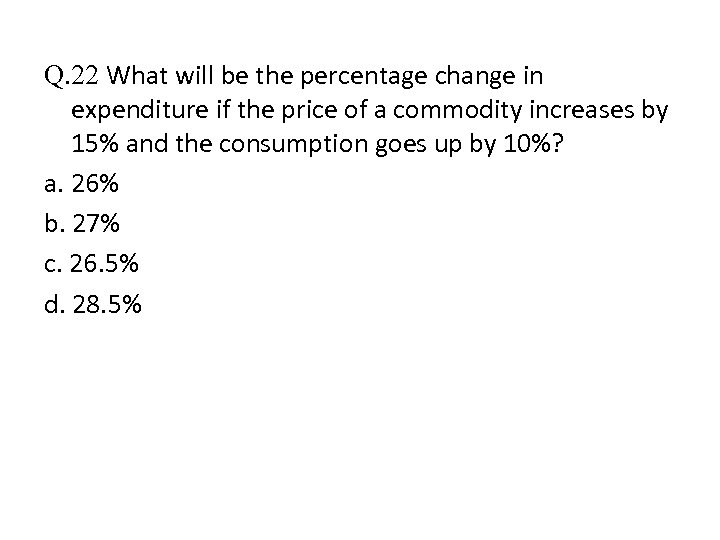Q. 22 What will be the percentage change in expenditure if the price of a commodity increases by 15% and the consumption goes up by 10%? a. 26% b. 27% c. 26. 5% d. 28. 5%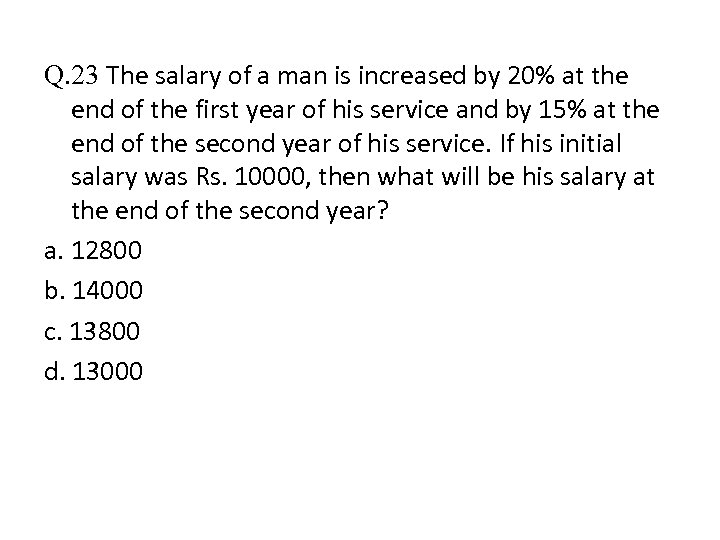Q. 23 The salary of a man is increased by 20% at the end of the first year of his service and by 15% at the end of the second year of his service. If his initial salary was Rs. 10000, then what will be his salary at the end of the second year? a. 12800 b. 14000 c. 13800 d. 13000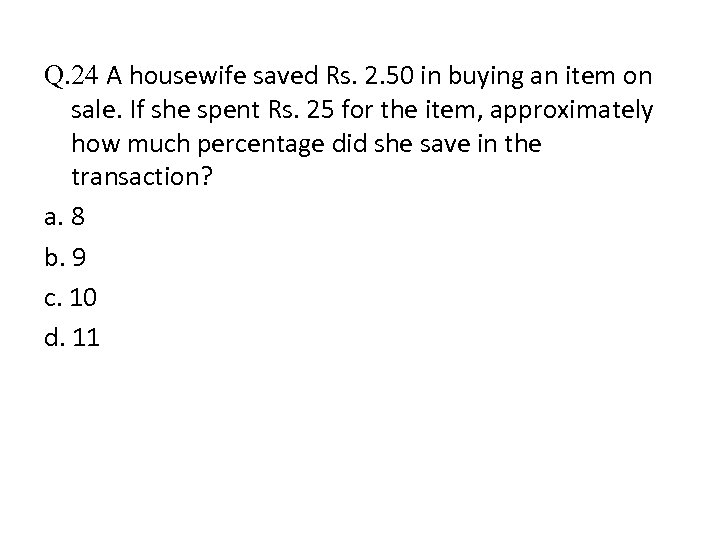Q. 24 A housewife saved Rs. 2. 50 in buying an item on sale. If she spent Rs. 25 for the item, approximately how much percentage did she save in the transaction? a. 8 b. 9 c. 10 d. 11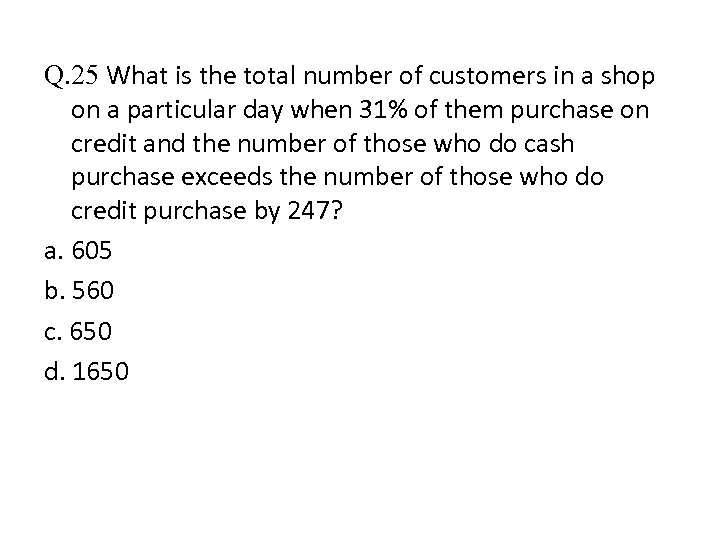Q. 25 What is the total number of customers in a shop on a particular day when 31% of them purchase on credit and the number of those who do cash purchase exceeds the number of those who do credit purchase by 247? a. 605 b. 560 c. 650 d. 1650